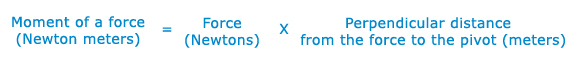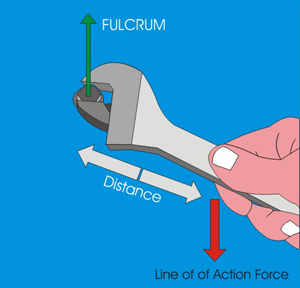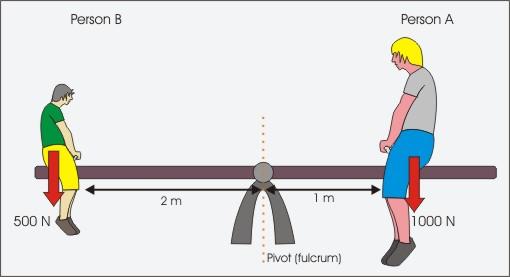## Moment

The turning effect of a force is known as the moment. It is the product of the force multiplied by the perpendicular distance from the line of action of the force to the pivot or point where the object will turn.When undoing a nut fastened to a screw by hand one realises that the amount of force required is a lot greater than when undoing the same nut using a spanner. The spanner increases the distance between the fulcrum and the line of action of the force, thus for the same force a greater moment is obtained.SMALL MOMENT The distance from the fulcrum to the line of action of force is very small LARGE MOMENT The distance from the fulcrum to the line of action of force is large

## Principle of Moments

The principle of moments states that when in equilibrium the total sum of the anti clockwise moment is equal to the total sum of the clockwise moment.

When a system is stable or balance it is said to be in equilibrium as all the forces acting on the system cancel each other out.

In equilibrium

Total Anticlockwise Moment = Total Clockwise Moment

This principle can be explained by considering two people on a seesaw.### Moments Acting On A Seesaw

Both people exert a downward force on the seesaw due to their weights.

Person A’s weight is trying to turn the seesaw anticlockwise whilst person B’s weight is trying to turn the seesaw clockwise.

Person A’s Moment = Force x perpendicular distance from fulcrum

1000  x 1  = 1000 Nm

Person B’s Moment = Force x perpendicular distance from fulcrum

500   x 2   = 1000 Nm

Persons A’s moment  = Persons B’s Moment
Anticlockwise moment = Clockwise moment

Therefore seesaw is in equilibrium.